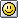## 2018年10月28日

### 数を味わう竜のウェイブスペル

ツォルキンが新しいサイクルに入って３日目。
KIN1の赤い磁気の竜から始まる竜のウェイブスペルの１３日間は、銀河の音＝太陽の紋章コードになる唯一の期間だ。

１：　未分化・ワンネス、生まれたありのままを育む竜
Unity, a unit, 1 kin, God as One, one which is in all number, Fibonacci 1 and 2, V21 H21 first coordinate of unity, initiates first, outermost circuit, 441 matrix

２：　分化・極性、スピリットの呼吸を伝える風
Polarity, binary power, basis of all even number, law of alternation, yin and yang, inhalation-exhalation, male-female, night-and-day etc. Fibonacci 3rd sequence

３：　関係性・奉仕、豊かさの直観を夢見る夜
Activation, rhythm, triplet factor, law of 3, root of all dynamism and synthesis, first 2-D form – triangle, triangular of 2, Fibonaccci 4th sequence

４：　形・周期、開花を目指す気づきの種
Form, measure, 2^2, square and all four cornered forms, definition of space (four primary directions), stages of primary cosmic cycle, harmonic, first 3-D form, tetrahedron

５：　倍音・輝き、命の本能を生き残らせる蛇
Fifth force, quintessence, pentacle, pentagon (72˚ of a circle, 73 days of one 365 day ring/year), a chromatic, basis of pentatonic scale, Fibonnaci 5th sequence

６：　リズム・バランス、死の機会を等しくする世界の橋渡し
Second platonic solid – Cube (six squares), Hexagon, basis of hexagisimal (count by sixes) code, snowflakes and crystal structures, basis of system of sixes as cosmic ordering principle, two triangles joined = tantric energy, triangular of 3, perfect number: 1+2+3 = 6, 1x2x3 = 6

７：　共鳴・調律、癒やしの遂行を知る手
Interval frequency of lost time in eternity, basis of manifest universe, resonant power of primal creation, mystic root, basis of heptad (seven –day sequence), root of 441, supreme power of Divine order; with 13, occulted basis of Cosmic Wheel of Law of Time 13:7  as sum = totality 20, key term of creation formula 4:7::7:13 reciprocal = 142857, perfect recombinant for first six multiples

８：　銀河・調和、芸術の気品を美しくする星
2 x 4, 2^3, Galactic form and order, octagon, octave (resonant fractal of whole), diatonic scale, basis of 3rd 3-d solid, octahedron Fibannaci 6th sequence

９：　太陽・意図、水の流れを清める月
Power number of rhythmic frequency of time – 3², universally consistent factors of 9 in decimal code always add to 9 or a multiple of 9, reciprocal = .111111 (see reciprocal of 11), basis of matrix of nine and of interval frequency of all numbers and their reverses as well as triplet series, number of Bolontiku – Nine Lords of Time

10：　地上・現実、忠実なハートを愛する犬
2 x 5, basis of decimal(increase by powers of ten) code, power of manifestation, triangular of 4, one half of totality (20)

11：　分光・手放し、魔術の幻想を遊ぶ猿
Prime key, mystic frequency, the magician, liberation, decimal analog to vigesimal 21, reciprocal = .090909, with 9 perfect cyclical recombinant, V21-H11 first gate left hand unity, 441 matrix

12：　水晶・協力、知恵の自由意志を感化する人
2 x 6, 4 x 3, organizational form, stasis, root of 144 key sacred formal frequency, 12 = reverse of 21 viz. 12/21 (2012), number of synodic lunations in one solar ring (one lunar year, Muslim calendar), 4th solid, dodecahedron

13：　宇宙・ここ、気づきの空間を探る空歩く者
Prime key, cyclic wholeness, Basis of wavespell, cosmic time fractal, frequency of cosmic time 13 x 20 (totality) = 260 galactic matrix = 13:20 frequency of time as universal factor of synchronization, with 7, basis of cosmic wheel and cosmology of time – 13:7 = 13:20 frequency, reverse of 31, viz. 3113 beginning of 13 baktun cycle, 13^2 = 169, reverse of 961 = 31^2, number of Oxlahuntiku, 13 lords of heavenly order, reciprocal = .076923, chromatic octave, Fibonacci 7th sequence, coordinating frequency first time dimension, cosmic creation

（英文は、時間の法則財団のサイトにある数441の辞典より　http://www.lawoftime.org/lawoftime/synchronotron-441-number-dictionary.html

waiar56 at 18:25コメント(0)１３の月の暦

### コメントする

名前 情報を記憶 評価 -- 1（最低） 2 3 4 5（最高） 顔 星プロフィール

カテゴリ別アーカイブ

ペット